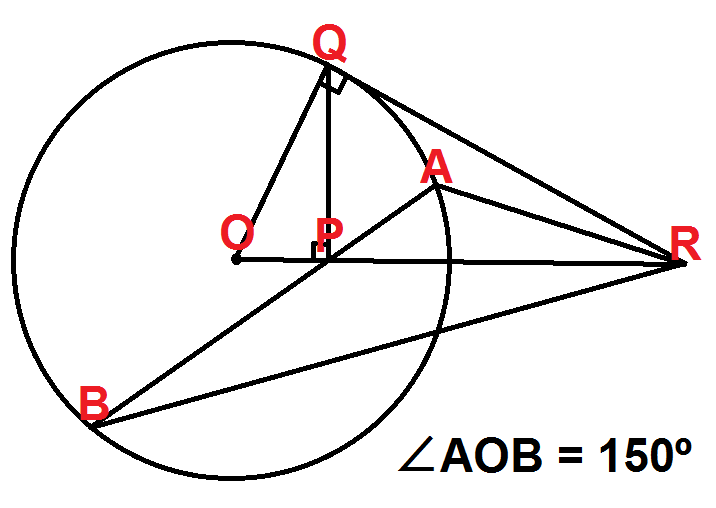# Day 3: Inverted Thinking

Geometry Level 5Let $O$ be the centre of a circle and let $P$ be a point inside this circle. Draw the radius $OQ$ such that $\angle OPQ$ is a right angle. Let $R$ be the intersection of the line $OP$ and of the tangent at $Q$. Let $AB$ be a chord passing through $P$ of the circle.

Given that $\angle AOB = 150^{\circ}$, find the value of $\angle ARP$.

This problem is part of the set Advent Calendar 2014.

×

Problem Loading...

Note Loading...

Set Loading...